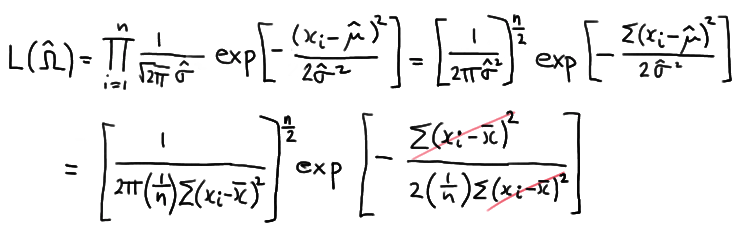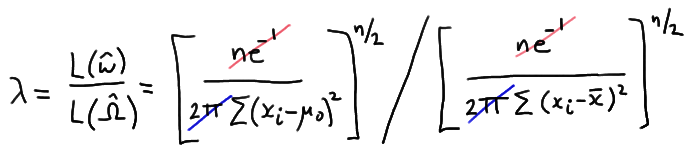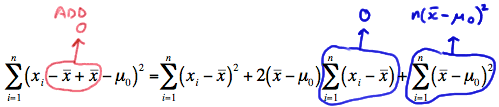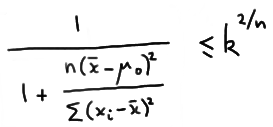# 27.2 - The T-Test For One Mean

27.2 - The T-Test For One Mean

Well, geez, now why would we be revisiting the t-test for a mean $$\mu$$ when we have already studied it back in the hypothesis testing section? Well, the answer, it turns out, is that, as we'll soon see, the t-test for a mean $$\mu$$ is the likelihood ratio test! Let's take a look!

## Example 27-4Suppose that a random sample $$X_1 , X_2 , \dots , X_n$$ arises from a normal population with unknown mean $$\mu$$ and unknown variance $$\sigma^2$$. (Yes, back to the realistic situation, in which we don't know the population variance either.) Find the size $$\alpha$$ likelihood ratio test for testing the null hypothesis $$H_0: \mu = \mu_0$$ against the two-sided alternative hypothesis $$H_A: \mu ≠ \mu_0$$.

Our unrestricted parameter space is:

$$\Omega = \left\{ (\mu, \sigma^2) : -\infty < \mu < \infty, 0 < \sigma^2 < \infty \right\}$$

Under the null hypothesis, the mean $$\mu$$ is the only parameter that is restricted. Therefore, our parameter space under the null hypothesis is:

$$\omega = \left\{(\mu, \sigma^2) : \mu =\mu_0, 0 < \sigma^2 < \infty \right\}$$

Now, first consider the case where the mean and variance are unrestricted. We showed back when we studied maximum likelihood estimation that the maximum likelihood estimates of $$\mu$$ and $$\sigma^2$$ are, respectively:

$$\hat{\mu} = \bar{x} \text{ and } \hat{\sigma}^2 = \dfrac{1}{n}\sum_{i=1}^{n}(x_i - \bar{x})^2$$

Therefore, the maximum of the likelihood function for the unrestricted parameter space is:which simplifies to:

$$L(\hat{\Omega})= \left[\dfrac{ne^{-1}}{2\pi \Sigma (x_i - \bar{x})^2} \right]^{n/2}$$

Now, under the null parameter space, the maximum likelihood estimates of $$\mu$$ and $$\sigma^2$$ are, respectively:

$$\hat{\mu} = \mu_0 \text{ and } \hat{\sigma}^2 = \dfrac{1}{n}\sum_{i=1}^{n}(x_i - \mu_0)^2$$

Therefore, the likelihood under the null hypothesis is:which simplifies to:

$$L(\hat{\omega})= \left[\dfrac{ne^{-1}}{2\pi \Sigma (x_i - \mu_0)^2} \right]^{n/2}$$

And now taking the ratio of the two likelihoods, we get:which reduces to:

$$\lambda = \left[ \dfrac{\sum_{i=1}^{n}(x_i - \bar{x})^2}{\sum_{i=1}^{n}(x_i - \mu_0)^2} \right] ^{n/2}$$

Focusing only on the denominator for a minute, let's do that trick again of "adding 0" in just the right away. Adding 0 to the quantity in the parentheses, we get:which simplifies to:

$$\sum_{i=1}^{n}(x_i - \mu_0)^2 = \sum_{i=1}^{n}(x_i - \bar{x})^2 +n(\bar{x} - \mu_0)^2$$

Then, our likelihood ratio $$\lambda$$ becomes:

$$\lambda = \left[ \dfrac{\sum_{i=1}^{n}(x_i - \bar{x})^2}{\sum_{i=1}^{n}(x_i - \mu_0)^2} \right] ^{n/2} = \left[ \dfrac{\sum_{i=1}^{n}(x_i - \bar{x})^2}{ \sum_{i=1}^{n}(x_i - \bar{x})^2 +n(\bar{x} - \mu_0)^2} \right] ^{n/2}$$

which, upon dividing through numerator and denominator by $$\sum_{i=1}^{n}(x_i - \bar{x})^2$$ simplifies to:Therefore, the likelihood ratio test's critical region, which is given by the inequality $$\lambda ≤ k$$, is equivalent to:which with some minor algebraic manipulation can be shown to be equivalent to:So, in a nutshell, we've shown that the likelihood ratio test tells us that for this situation we should reject the null hypothesis $$H_0: \mu= \mu_0$$ in favor of the alternative hypothesis $$H_A: \mu ≠ \mu_0$$ if:

$$\dfrac{(\bar{x}-\mu_0)^2 }{s^2 / n} \ge k^{*}$$

Well, okay, so I started out this page claiming that the t-test for a mean $$\mu$$ is the likelihood ratio test. Is it? Well, the above critical region is equivalent to rejecting the null hypothesis if:

$$\dfrac{|\bar{x}-\mu_0| }{s / \sqrt{n}} \ge k^{**}$$

Does that look familiar? We previously learned that if $$X_1, X_2, \dots, X_n$$ are normally distributed with mean $$\mu$$ and variance $$\sigma^2$$, then:

$$T = \dfrac{\bar{X}-\mu}{S / \sqrt{n}}$$

follows a T distribution with n − 1 degrees of freedom. So, this tells us that we should use the T distribution to choose $$k^{**}$$. That is, set:

$$k^{**} = t_{\alpha /2, n-1}$$

and we have our size $$\alpha$$ t-test that ensures the probability of committing a Type I error is $$\alpha$$.

It turns out... we didn't know it at the time... but every hypothesis test that we derived in the hypothesis testing section is a likelihood ratio test. Back then, we derived each test using distributional results of the relevant statistic(s), but we could have alternatively, and perhaps just as easily, derived the tests using the likelihood ratio testing method.

  Link ↥ Has Tooltip/Popover Toggleable Visibility